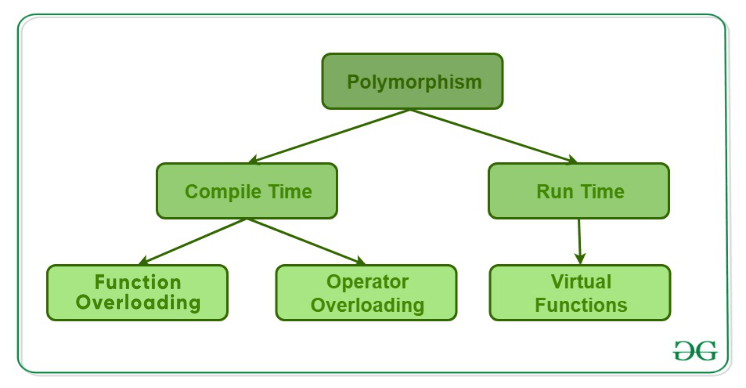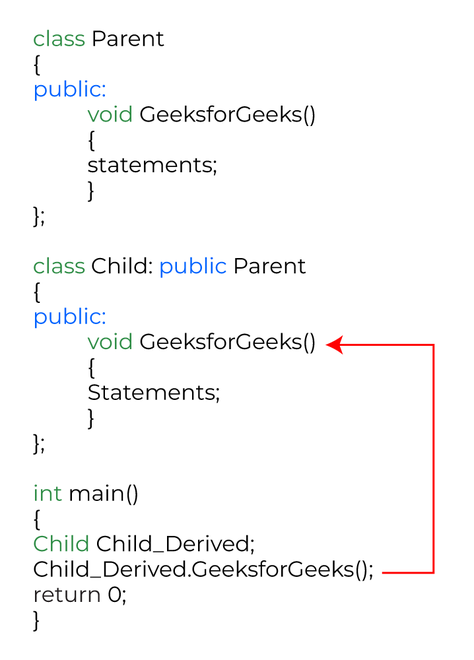Open In App
Related Articles
• Write an Interview Experience
• C++ Programming Language

# C++ Polymorphism

The word “polymorphism” means having many forms. In simple words, we can define polymorphism as the ability of a message to be displayed in more than one form. A real-life example of polymorphism is a person who at the same time can have different characteristics. A man at the same time is a father, a husband, and an employee. So the same person exhibits different behavior in different situations. This is called polymorphism. Polymorphism is considered one of the important features of Object-Oriented Programming.

## Types of Polymorphism

• Compile-time Polymorphism
• Runtime PolymorphismTypes of Polymorphism

## 1. Compile-Time Polymorphism

When there are multiple functions with the same name but different parameters, then the functions are said to be overloaded, hence this is known as Function Overloading. Functions can be overloaded by changing the number of arguments or/and changing the type of arguments. In simple terms, it is a feature of object-oriented programming providing many functions that have the same name but distinct parameters when numerous tasks are listed under one function name. There are certain Rules of Function Overloading that should be followed while overloading a function.

Below is the C++ program to show function overloading or compile-time polymorphism:

## C++

 `// C++ program to demonstrate``// function overloading or``// Compile-time Polymorphism``#include ` `using` `namespace` `std;``class` `Geeks {``public``:``    ``// Function with 1 int parameter``    ``void` `func(``int` `x)``    ``{``        ``cout << ``"value of x is "` `<< x << endl;``    ``}` `    ``// Function with same name but``    ``// 1 double parameter``    ``void` `func(``double` `x)``    ``{``        ``cout << ``"value of x is "` `<< x << endl;``    ``}` `    ``// Function with same name and``    ``// 2 int parameters``    ``void` `func(``int` `x, ``int` `y)``    ``{``        ``cout << ``"value of x and y is "` `<< x << ``", "` `<< y``             ``<< endl;``    ``}``};` `// Driver code``int` `main()``{``    ``Geeks obj1;` `    ``// Function being called depends``    ``// on the parameters passed``    ``// func() is called with int value``    ``obj1.func(7);` `    ``// func() is called with double value``    ``obj1.func(9.132);` `    ``// func() is called with 2 int values``    ``obj1.func(85, 64);``    ``return` `0;``}`

Output

```value of x is 7
value of x is 9.132
value of x and y is 85, 64```

Explanation: In the above example, a single function named function func() acts differently in three different situations, which is a property of polymorphism. To know more about this, you can refer to the article – Function Overloading in C++.

C++ has the ability to provide the operators with a special meaning for a data type, this ability is known as operator overloading. For example, we can make use of the addition operator (+) for string class to concatenate two strings. We know that the task of this operator is to add two operands. So a single operator ‘+’, when placed between integer operands, adds them and when placed between string operands, concatenates them.

## CPP

 `// C++ program to demonstrate``// Operator Overloading or``// Compile-Time Polymorphism``#include ``using` `namespace` `std;` `class` `Complex {``private``:``    ``int` `real, imag;` `public``:``    ``Complex(``int` `r = 0, ``int` `i = 0)``    ``{``        ``real = r;``        ``imag = i;``    ``}` `    ``// This is automatically called``    ``// when '+' is used with between``    ``// two Complex objects``    ``Complex operator+(Complex ``const``& obj)``    ``{``        ``Complex res;``        ``res.real = real + obj.real;``        ``res.imag = imag + obj.imag;``        ``return` `res;``    ``}``    ``void` `print() { cout << real << ``" + i"` `<< imag << endl; }``};` `// Driver code``int` `main()``{``    ``Complex c1(10, 5), c2(2, 4);` `    ``// An example call to "operator+"``    ``Complex c3 = c1 + c2;``    ``c3.print();``}`

Output

`12 + i9`

Explanation:  In the above example, the operator ‘+’ is overloaded. Usually, this operator is used to add two numbers (integers or floating point numbers), but here the operator is made to perform the addition of two imaginary or complex numbers. To know more about this one, refer to the article – Operator Overloading.

## 2. Runtime Polymorphism

This type of polymorphism is achieved by Function Overriding. Late binding and dynamic polymorphism are other names for runtime polymorphism. The function call is resolved at runtime in runtime polymorphism. In contrast, with compile time polymorphism, the compiler determines which function call to bind to the object after deducing it at runtime.

### A. Function Overriding

Function Overriding occurs when a derived class has a definition for one of the member functions of the base class. That base function is said to be overridden.Function overriding Explanation

#### Runtime Polymorphism with Data Members

Runtime Polymorphism can be achieved by data members in C++. Let’s see an example where we are accessing the field by reference variable which refers to the instance of the derived class.

## C++

 `// C++ program for function overriding with data members``#include ``using` `namespace` `std;` `//  base class declaration.``class` `Animal {``public``:``    ``string color = ``"Black"``;``};` `// inheriting Animal class.``class` `Dog : ``public` `Animal {``public``:``    ``string color = ``"Grey"``;``};` `// Driver code``int` `main(``void``)``{``    ``Animal d = Dog(); ``// accessing the field by reference``                      ``// variable which refers to derived``    ``cout << d.color;``}`

Output

`Black`

### B. Virtual Function

A virtual function is a member function that is declared in the base class using the keyword virtual and is re-defined (Overridden) in the derived class.

#### Some Key Points About Virtual Functions:

• Virtual functions are Dynamic in nature.
• They are defined by inserting the keyword “virtual” inside a base class and are always declared with a base class and overridden in a child class
• A virtual function is called during Runtime

Below is the C++ program to demonstrate virtual function:

## C++

 `// C++ Program to demonstrate``// the Virtual Function``#include ``using` `namespace` `std;` `// Declaring a Base class``class` `GFG_Base {` `public``:``    ``// virtual function``    ``virtual` `void` `display()``    ``{``        ``cout << ``"Called virtual Base Class function"``             ``<< ``"\n\n"``;``    ``}` `    ``void` `print()``    ``{``        ``cout << ``"Called GFG_Base print function"``             ``<< ``"\n\n"``;``    ``}``};` `// Declaring a Child Class``class` `GFG_Child : ``public` `GFG_Base {` `public``:``    ``void` `display()``    ``{``        ``cout << ``"Called GFG_Child Display Function"``             ``<< ``"\n\n"``;``    ``}` `    ``void` `print()``    ``{``        ``cout << ``"Called GFG_Child print Function"``             ``<< ``"\n\n"``;``    ``}``};` `// Driver code``int` `main()``{``    ``// Create a reference of class GFG_Base``    ``GFG_Base* base;` `    ``GFG_Child child;` `    ``base = &child;` `    ``// This will call the virtual function``    ``base->GFG_Base::display();` `    ``// this will call the non-virtual function``    ``base->print();``}`

Output

```Called virtual Base Class function

Called GFG_Base print function```

Example 2:

## C++

 `// C++ program for virtual function overriding``#include ``using` `namespace` `std;` `class` `base {``public``:``    ``virtual` `void` `print()``    ``{``        ``cout << ``"print base class"` `<< endl;``    ``}` `    ``void` `show() { cout << ``"show base class"` `<< endl; }``};` `class` `derived : ``public` `base {``public``:``    ``// print () is already virtual function in``    ``// derived class, we could also declared as``    ``// virtual void print () explicitly``    ``void` `print() { cout << ``"print derived class"` `<< endl; }` `    ``void` `show() { cout << ``"show derived class"` `<< endl; }``};` `// Driver code``int` `main()``{``    ``base* bptr;``    ``derived d;``    ``bptr = &d;` `    ``// Virtual function, binded at``    ``// runtime (Runtime polymorphism)``    ``bptr->print();` `    ``// Non-virtual function, binded``    ``// at compile time``    ``bptr->show();` `    ``return` `0;``}`

Output

```print derived class
show base class```